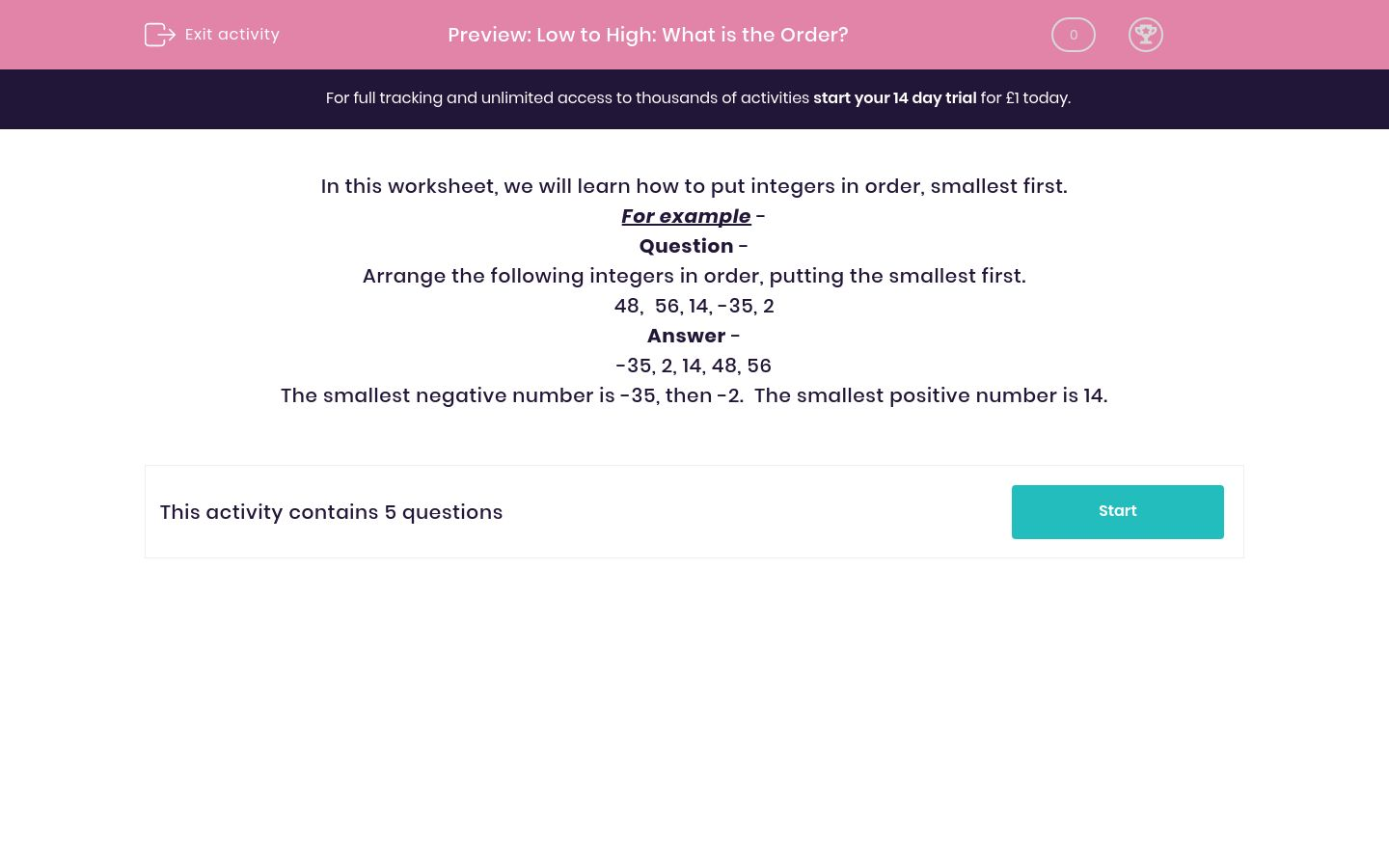# Low to High: What is the Order?

In this worksheet, students arrange positive and negative integers in increasing order of size.Key stage:  KS 2

Curriculum topic:   Maths and Numerical Reasoning

Curriculum subtopic:   Place Value

Difficulty level:### QUESTION 1 of 10

In this worksheet, we will learn how to put integers in order, smallest first.

For example -

Question -

Arrange the following integers in order, putting the smallest first.

48,  56, 14, -35, 2

-35, 2, 14, 48, 56

The smallest negative number is -35, then -2.  The smallest positive number is 14.

---- OR ----